# Seaborn的6个简单技巧#### 基线图

# 导入包
import matplotlib.pyplot as plt
import seaborn as sns

# 导入数据
df# 图
sns.scatterplot(data=df, x='body_mass_g', y='bill_length_mm',
alpha=0.7, hue='species', size='gender')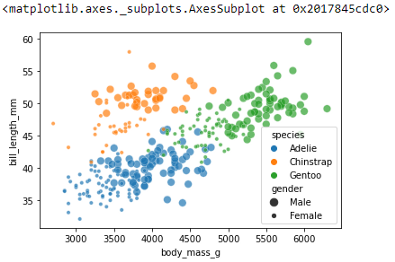#### 技巧1：分号

# 图
sns.scatterplot(data=df, x='body_mass_g', y='bill_length_mm',
alpha=0.7, hue='species', size='gender');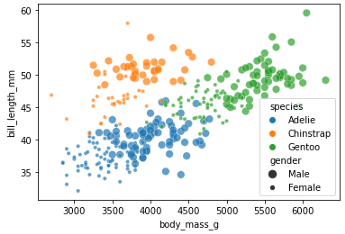#### 技巧2：plt.figure()

# 图
plt.figure(figsize=(9, 5))
sns.scatterplot(data=df, x='body_mass_g', y='bill_length_mm',
alpha=0.7, hue='species', size='gender');# 图
plt.figure(figsize=(9, 5))
sns.scatterplot(data=df, x='body_mass_g', y='bill_length_mm',
alpha=0.7, hue='species', size='gender')
plt.legend(loc='upper right', bbox_to_anchor=(1.2, 1));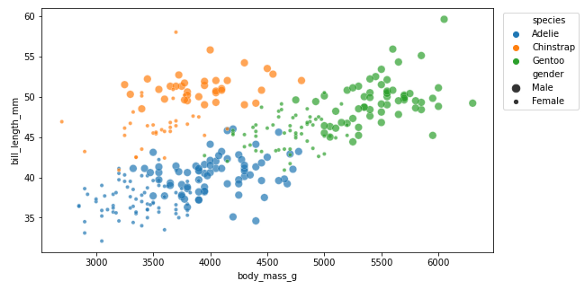#### 技巧3：sns.set_style()

# 更改默认样式
sns.set_style('whitegrid')

# 图
plt.figure(figsize=(9, 5))
sns.scatterplot(data=df, x='body_mass_g', y='bill_length_mm',
alpha=0.7, hue='species', size='gender')
plt.legend(loc='upper right', bbox_to_anchor=(1.2, 1));#### 技巧4：sns.set_context()

# 默认上下文更改
sns.set_context('talk')

# 图
plt.figure(figsize=(9, 5))
sns.scatterplot(data=df, x='body_mass_g', y='bill_length_mm',
alpha=0.7, hue='species', size='gender')
plt.legend(loc='upper right', bbox_to_anchor=(1.3, 1));#### 技巧5：sns.set_palette()

# 更改默认调色板
sns.set_palette('rainbow')

# 图
plt.figure(figsize=(9, 5))
sns.scatterplot(data=df, x='body_mass_g', y='bill_length_mm',
alpha=0.7, hue='species', size='gender')
plt.legend(loc='upper right', bbox_to_anchor=(1.3, 1));# 更改默认调色板
sns.set_palette(['green', 'purple', 'red'])

# 图
plt.figure(figsize=(9, 5))
sns.scatterplot(data=df, x='body_mass_g', y='bill_length_mm',
alpha=0.7, hue='species', size='gender')
plt.legend(loc='upper right', bbox_to_anchor=(1.3, 1));# 更改默认调色板
sns.set_palette(['#62C370', '#FFD166', '#EF476F'])

# 图
plt.figure(figsize=(9, 5))
sns.scatterplot(data=df, x='body_mass_g', y='bill_length_mm',
alpha=0.7, hue='species', size='gender')
plt.legend(loc='upper right', bbox_to_anchor=(1.3, 1));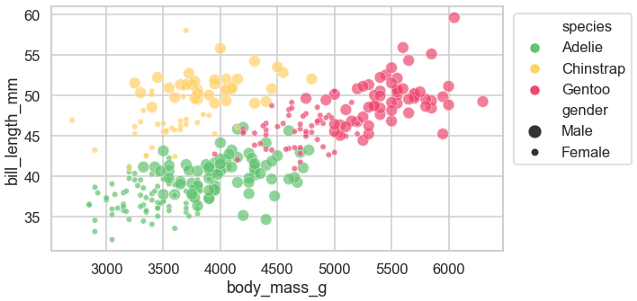#### 技巧6：sns.set()

# 导入包
import matplotlib.pyplot as plt
import seaborn as sns

# 更改默认值
sns.set_style('whitegrid')
sns.set_context('talk')
sns.set_palette('rainbow')

# 导入包
import matplotlib.pyplot as plt
import seaborn as sns

# 更改默认值
sns.set(style='whitegrid', context='talk', palette='rainbow')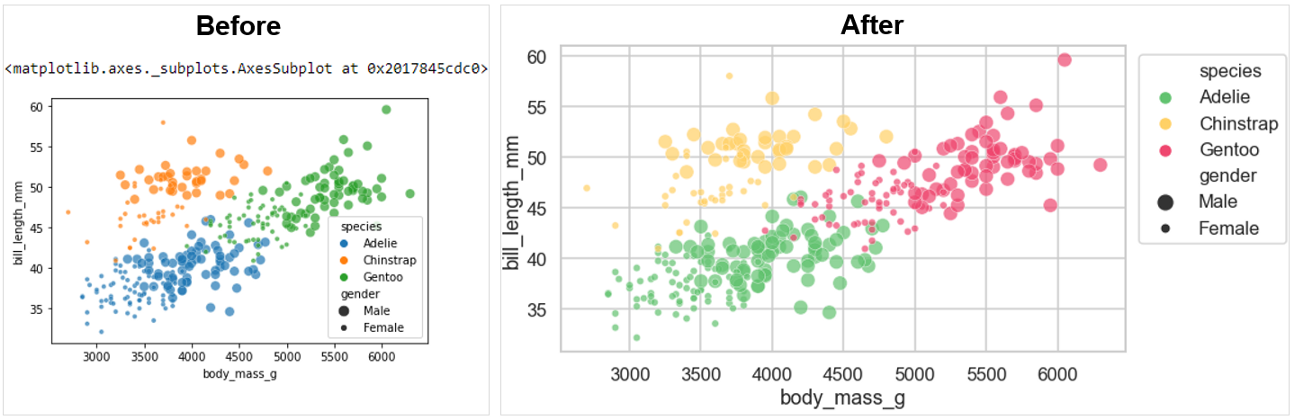http://panchuang.net/

sklearn机器学习中文官方文档：
http://sklearn123.com/

http://docs.panchuang.net/